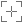# EPSG:1750

## NAD83 to WGS 84 (54)

### Attributes

Ellipsoid: GRS 1980

Prime meridian: Greenwich

Data source: EPSG

Information source: OGP

Revision date: 2021-09-10

Scope: (null/copy) Approximation for medium and low accuracy applications assuming equality between plate-fixed static and earth-fixed dynamic CRSs, ignoring static/dynamic CRS differences.

Remarks: Parameter files are from NAD83 to NAD83(HARN) (4) (code 1477) assuming that NAD83(HARN) is equivalent to WGS 84 within the accuracy of the transformation.

Area of use: United States (USA) - California south of 36.5°N

Description: (0,)United States (USA) - California south of 36.5°N

#### Export

Definition: PROJ.4

`+proj=pipeline +step +proj=axisswap +order=2,1 +step +proj=unitconvert +xy_in=deg +xy_out=rad +step +proj=hgridshift +grids=us_noaa_cshpgn.tif +step +proj=unitconvert +xy_in=rad +xy_out=deg +step +proj=axisswap +order=2,1`

Definition: JavaScript (Proj4js)

`proj4.defs("EPSG:1750","+proj=pipeline +step +proj=axisswap +order=2,1 +step +proj=unitconvert +xy_in=deg +xy_out=rad +step +proj=hgridshift +grids=us_noaa_cshpgn.tif +step +proj=unitconvert +xy_in=rad +xy_out=deg +step +proj=axisswap +order=2,1");`

Definition: JSON

```{
"\$schema": "https://proj.org/schemas/v0.4/projjson.schema.json",
"type": "Transformation",
"name": "NAD83 to WGS 84 (54)",
"source_crs": {
"type": "GeographicCRS",
"datum": {
"type": "GeodeticReferenceFrame",
"name": "North American Datum 1983",
"ellipsoid": {
"name": "GRS 1980",
"semi_major_axis": 6378137,
"inverse_flattening": 298.257222101
}
},
"coordinate_system": {
"subtype": "ellipsoidal",
"axis": [
{
"name": "Geodetic latitude",
"abbreviation": "Lat",
"direction": "north",
"unit": "degree"
},
{
"name": "Geodetic longitude",
"abbreviation": "Lon",
"direction": "east",
"unit": "degree"
}
]
},
"id": {
"authority": "EPSG",
"code": 4269
}
},
"target_crs": {
"type": "GeographicCRS",
"name": "WGS 84",
"datum_ensemble": {
"name": "World Geodetic System 1984 ensemble",
"members": [
{
"name": "World Geodetic System 1984 (Transit)",
"id": {
"authority": "EPSG",
"code": 1166
}
},
{
"name": "World Geodetic System 1984 (G730)",
"id": {
"authority": "EPSG",
"code": 1152
}
},
{
"name": "World Geodetic System 1984 (G873)",
"id": {
"authority": "EPSG",
"code": 1153
}
},
{
"name": "World Geodetic System 1984 (G1150)",
"id": {
"authority": "EPSG",
"code": 1154
}
},
{
"name": "World Geodetic System 1984 (G1674)",
"id": {
"authority": "EPSG",
"code": 1155
}
},
{
"name": "World Geodetic System 1984 (G1762)",
"id": {
"authority": "EPSG",
"code": 1156
}
},
{
"name": "World Geodetic System 1984 (G2139)",
"id": {
"authority": "EPSG",
"code": 1309
}
}
],
"ellipsoid": {
"name": "WGS 84",
"semi_major_axis": 6378137,
"inverse_flattening": 298.257223563
},
"accuracy": "2.0",
"id": {
"authority": "EPSG",
"code": 6326
}
},
"coordinate_system": {
"subtype": "ellipsoidal",
"axis": [
{
"name": "Geodetic latitude",
"abbreviation": "Lat",
"direction": "north",
"unit": "degree"
},
{
"name": "Geodetic longitude",
"abbreviation": "Lon",
"direction": "east",
"unit": "degree"
}
]
},
"id": {
"authority": "EPSG",
"code": 4326
}
},
"method": {
"id": {
"authority": "EPSG",
"code": 9613
}
},
"parameters": [
{
"name": "Latitude difference file",
"value": "cshpgn.las",
"id": {
"authority": "EPSG",
"code": 8657
}
},
{
"name": "Longitude difference file",
"value": "cshpgn.los",
"id": {
"authority": "EPSG",
"code": 8658
}
}
],
"accuracy": "2.0",
"scope": "(null/copy) Approximation for medium and low accuracy applications assuming equality between plate-fixed static and earth-fixed dynamic CRSs, ignoring static/dynamic CRS differences.",
"area": "United States (USA) - California south of 36.5°N",
"bbox": {
"south_latitude": 32.53,
"west_longitude": -121.98,
"north_latitude": 36.5,
"east_longitude": -114.12
},
"id": {
"authority": "EPSG",
"code": 1750
},
"remarks": "Parameter files are from NAD83 to NAD83(HARN) (4) (code 1477) assuming that NAD83(HARN) is equivalent to WGS 84 within the accuracy of the transformation."
}
```

Definition: MapServer - MAPfile

```PROJECTION
"proj=pipeline"
"step"
"proj=axisswap"
"order=2,1"
"step"
"proj=unitconvert"
"xy_in=deg"
"step"
"proj=hgridshift"
"grids=us_noaa_cshpgn.tif"
"step"
"proj=unitconvert"
"xy_out=deg"
"step"
"proj=axisswap"
"order=2,1"
END```

Definition: Mapnik

```<?xml version="1.0" encoding="utf-8"?>
<Map srs="+proj=pipeline +step +proj=axisswap +order=2,1 +step +proj=unitconvert +xy_in=deg +xy_out=rad +step +proj=hgridshift +grids=us_noaa_cshpgn.tif +step +proj=unitconvert +xy_in=rad +xy_out=deg +step +proj=axisswap +order=2,1">
<Layer srs="+proj=pipeline +step +proj=axisswap +order=2,1 +step +proj=unitconvert +xy_in=deg +xy_out=rad +step +proj=hgridshift +grids=us_noaa_cshpgn.tif +step +proj=unitconvert +xy_in=rad +xy_out=deg +step +proj=axisswap +order=2,1">
</Layer>
</Map>```

Definition: SQL (PostGIS)

`INSERT into spatial_ref_sys (srid, auth_name, auth_srid, proj4text, srtext) values ( 1750, 'EPSG', 1750, '+proj=pipeline +step +proj=axisswap +order=2,1 +step +proj=unitconvert +xy_in=deg +xy_out=rad +step +proj=hgridshift +grids=us_noaa_cshpgn.tif +step +proj=unitconvert +xy_in=rad +xy_out=deg +step +proj=axisswap +order=2,1', 'None');`

Definition: OGC WKT 2 (2019)

`COORDINATEOPERATION["NAD83 to WGS 84 (54)",VERSION["EPSG-Usa CA s"],SOURCECRS[GEOGCRS["NAD83",DATUM["North American Datum 1983",ELLIPSOID["GRS 1980",6378137,298.257222101,LENGTHUNIT["metre",1]]],PRIMEM["Greenwich",0,ANGLEUNIT["degree",0.0174532925199433]],CS[ellipsoidal,2],AXIS["geodetic latitude (Lat)",north,ORDER,ANGLEUNIT["degree",0.0174532925199433]],AXIS["geodetic longitude (Lon)",east,ORDER,ANGLEUNIT["degree",0.0174532925199433]],ID["EPSG",4269]]],TARGETCRS[GEOGCRS["WGS 84",ENSEMBLE["World Geodetic System 1984 ensemble",MEMBER["World Geodetic System 1984 (Transit)"],MEMBER["World Geodetic System 1984 (G730)"],MEMBER["World Geodetic System 1984 (G873)"],MEMBER["World Geodetic System 1984 (G1150)"],MEMBER["World Geodetic System 1984 (G1674)"],MEMBER["World Geodetic System 1984 (G1762)"],MEMBER["World Geodetic System 1984 (G2139)"],ELLIPSOID["WGS 84",6378137,298.257223563,LENGTHUNIT["metre",1]],ENSEMBLEACCURACY[2.0]],PRIMEM["Greenwich",0,ANGLEUNIT["degree",0.0174532925199433]],CS[ellipsoidal,2],AXIS["geodetic latitude (Lat)",north,ORDER,ANGLEUNIT["degree",0.0174532925199433]],AXIS["geodetic longitude (Lon)",east,ORDER,ANGLEUNIT["degree",0.0174532925199433]],ID["EPSG",4326]]],METHOD["NADCON",ID["EPSG",9613]],PARAMETERFILE["Latitude difference file","cshpgn.las"],PARAMETERFILE["Longitude difference file","cshpgn.los"],OPERATIONACCURACY[2.0],USAGE[SCOPE["(null/copy) Approximation for medium and low accuracy applications assuming equality between plate-fixed static and earth-fixed dynamic CRSs, ignoring static/dynamic CRS differences."],AREA["United States (USA) - California south of 36.5°N"],BBOX[32.53,-121.98,36.5,-114.12]],ID["EPSG",1750],REMARK["Parameter files are from NAD83 to NAD83(HARN) (4) (code 1477) assuming that NAD83(HARN) is equivalent to WGS 84 within the accuracy of the transformation."]]`